• 由伪微分算子启发的卷积神经网络用于有限角度层析成像重建 许可证： GNU通用公共许可证v3.0 作者： Mathilde Galinier 院校：摩德纳与雷焦艾米利亚大学 博士课程：由Marie Sklodowska-Curie Actions共同资助的INdAM...
• 用于2D卷积神经网络的Matlab代码 受到“”和“”的启发，但以教育为目的。 提供精心设计的matlab类层次结构，通过简单地阅读代码，可以帮助人们了解卷积神经网络和多层感知器（MLP）的工作流程。 概括： 基本层（M到...
• 用于2D卷积神经网络的Matlab代码 受到“”和“”的启发，但以教育为目的。 提供精心设计的matlab类层次结构，通过简单地阅读代码，可以帮助人们了解卷积神经网络和多层感知器（MLP）的工作流程。 概括： 基本层（M到...
• 文章目录1. 前言2. 全连接BP神经网络的缺点3. 卷积神经网络基本单元3.1 卷积（Convolution）3.2 ... 卷积神经网络的反向传播过程5.1 卷积层的误差传5.2 池化层的误差传6. 代码实现6.1 Numpy实现6.1.1 卷积层前...
文章目录1. 前言2. 全连接BP神经网络的缺点3. 卷积神经网络基本单元3.1 卷积（Convolution）3.2 填充（Padding）和步长（Stride）3.3 完整的卷积过程3.4 池化（Pooling)3.5 激活函数4. 卷积神经网络的前向传播过程5. 卷积神经网络的反向传播过程5.1 卷积层的误差反传5.2 池化层的误差反传6. 代码实现6.1 Numpy实现6.1.1 卷积层前向传播6.1.2 卷积层反向传播6.1.3 池化前向传播6.1.4 池化反向传播6.2 Tensorflow框架实现7. 一些优化方法8. 后记参考资料：
1. 前言
​	我之前写过一篇关于BP神经网络原理的文章，主要内容包括：BP神经网络的反向传播原理，以及简单的代码实现。本文和《BP 神经网络入门：从原理到应用》一文一脉相承，因此，我希望读者务必先看看这篇文章（链接在这里：BP 神经网络入门：从原理到应用,
​	好多读者都说，之前那篇BP神经网络的文章，公式太多，阅读体验不好，所以本文我将尽量减少公式，加入更多的图解和通俗解释。
​	本文主要包括以下内容：

卷积神经网络结构

前向传播原理

反向传播原理

代码实现

模型测试

简单的优化设计方法；优化设计方法有很多，本文不做过多介绍，如果有必要，我会后面会写个有关优化设计的课程。

2. 全连接BP神经网络的缺点
​	像全连接BP神经网络一样，卷积神经网络，也有一定的生物学基础（虽然，有时候确实感觉它的生物学解释有些牵强）。
​	我在BP 神经网络入门：从原理到应用一文，实现了一个，识别图片是不是猫的程序。在这个程序里，我们把输入的图片“展平”了，“展平”是什么意思呢？我们都知道，计算机存储图片，实际是存储了一个$W\times H\times D$ 的数组（W，H，D分别表示宽，高和维数，彩色图片包含RGB三维），如下图所示，“展平”就是，把这个数组变成一列，然后，输入神经网络进行训练：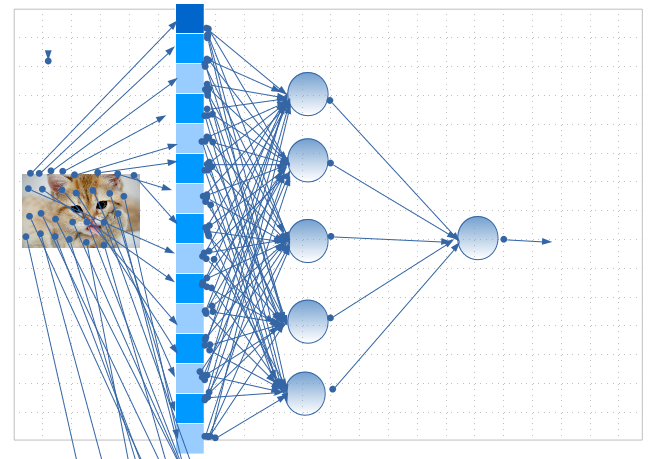​	这种方法，在图片尺寸较小的时候，还是可以接受的；但是，当图片尺寸比较大的时候，由于图像的像素点很多，神经网络的  层与层之间  的连接数量，会爆炸式增长，大大降低网络的性能。
​	除此之外，把图片数据展开成一列，破坏了图片的空间信息，试想，如果把上图中的猫图片变成一列，再把这一列数据，以图片的形式展示给你，你还能看出这表示一只猫吗？并且，有相关研究表明，人类大脑，在理解图片信息的过程中，并不是同时观察整个图片，而是更倾向于，观察部分特征，然后根据特征匹配、组合，得出整图信息。以下图为例：​	在这张图片中，你只能看到，猫的鼻子，1只眼睛，2只耳朵和嘴，虽然没有尾巴，爪子等其它信息，但是，你依然可以判断，这里有一只猫。所以说，人在理解图像信息的过程中，更专注于，局部特征，以及这些特征之间的组合。
​	换句话说，在BP全连接神经网络中，隐含层每一个神经元，都对输入图片  每个像素点  做出反应。这种机制包含了太多冗余连接。为了减少这些冗余，只需要每个隐含神经元，对图片的一小部分区域，做出反应就好了！卷积神经网络，正是基于这种想法而实现的。
3. 卷积神经网络基本单元
3.1 卷积（Convolution）
​	如果，你学过信号处理，或者有相关专业的数学基础，可能需要注意一下，这里的“卷积”，和信号处理里的卷积，不太一样，虽然，在数学表达式形式上有一点类似。
​	在卷积神经网络中，“卷积”更像是一个，特征提取算子。什么是特征提取算子呢？简单来说就是，提取图片纹理、边缘等特征信息的滤波器。下面，举个简单的例子，解释一下 边缘 特征提取算子是怎么工作的：​	比如有一张猫图片，人类在理解这张图片的时候，可能观察到圆圆的眼睛，可爱的耳朵，于是，判断这是一只猫。但是，机器怎么处理这个问题呢？传统的计算机视觉方法，通常设计一些算子（特征提取滤波器），来找到比如眼睛的边界，耳朵的边界，等信息，然后综合这些特征，得出结论——这是一只猫。
​	具体是怎么做的呢：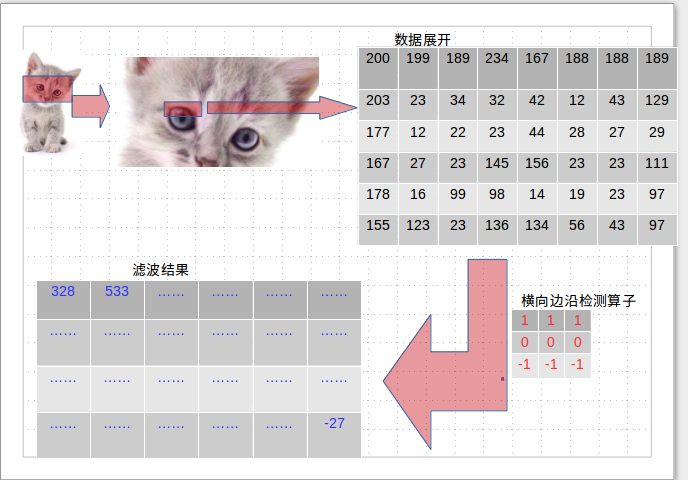​	如上图所示，假设，猫的上眼皮部分（框出部分），这部分的数据，展开后如图中数组所示**（这里，为了叙述简便，忽略了rgb三维通道，把图像当成一个二维数组[类比灰度图片]），我们使用一个算子，来检测横向边沿**——这个算子，让第一行的值减去第三行的值，得出结果。具体计算过程如下，比如，原图左上角数据，经过这个算子：
$200\times1+199\times1+189\times1+203\times0+23\times0+34\times0+177\times(-1)+12\times(-1)+22\times(-1)=328$
​	然后，算子向右滑动一个像素，得到第二个输出：533，依次向右滑动，最终得到第一行输出；
​	然后，向下滑动一个像素，到达第二行；在第二行，从左向右滑动，得到第二行输出……
​	动图演示过程如下（图片来自csdn）：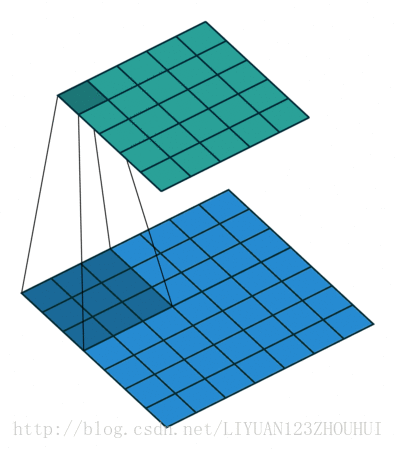​	从输出结果可以看出，在上眼皮处颜色较深，而眼皮上方和下方，无论是眼皮上方的毛发，还是眼皮下方的眼白，颜色都很浅，因此，这样做之后，如果滤波结果中有大值出现，就意味着，这里出现了横向边沿。不断在原图上滑动这个算子，我们就可以，检测到图像中所有的横向边沿。传统的机器视觉方法，就是设计更多更复杂的算子，检测更多的特征，最后，组合这些特征，得出判断结果。
​	卷积神经网络，做了类似的事情，所谓卷积，就是把一个算子在原图上不断滑动，得出滤波结果——这个结果，我们叫做“特征图”（Feature Map），这些算子被称为“卷积核”(Convolution Kernel)。不同的是，我们不必人工设计这些算子，而是使用随机初始化，来得到很多卷积核（算子），然后通过反向传播，优化这些卷积核，以期望得到更好的识别结果。
3.2 填充（Padding）和步长（Stride）
​	你可能注意到了，刚刚，我们“滑动”卷积核之后，输出变小了；具体来说，猫眼睛部分数据，尺寸是$6\times 8$，但是，经过一个$3\times 3$的卷积核之后，变成了$4\times 6$，这是因为卷积核有尺寸，如下图所示，当卷积核(橙色)滑动到边界时，就无法向右继续滑动了：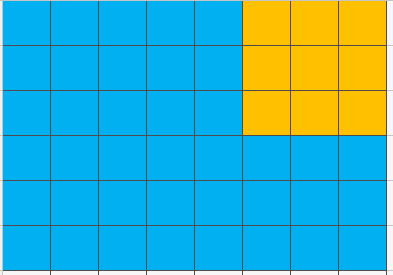​	可想而知，如果经过很多层卷积的话，输出尺寸会变的很小，同时图像边缘信息，会迅速流失，这对模型的性能，有着不可忽视的影响。为了减少卷积操作导致的，边缘信息丢失，我们需要进行填充（Padding），即在原图周围，添加一圈值为“0”的像素点（zero padding），这样的话，输出维度就和输入维度一致了。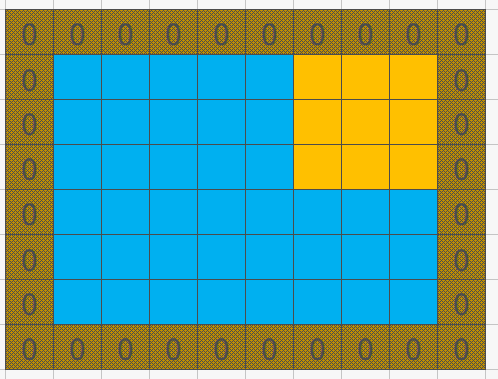​	还有一个很重要的因素是，步长（Stride），即卷积核每次滑动几个像素。前面，我们默认，卷积核每次滑动一个像素，其实也可以，每次滑动两个像素。其中，每次滑动的像素数，称为“步长”。
​	你可能会有疑问，如果步长大于 1 ，那不也会造成输出尺寸变小吗？是的！但是这种情况下，不会“故意”丢失边缘信息，即使有信息丢失，也是在整张图片上，比较“温和”地，舍弃了一些无关紧要的信息（反向传播调节卷积核参数，使之“智能”地舍弃无关信息）。但是，不能频繁使用步长为2。因为，如果输出尺寸变得，过小的话，即使卷积核参数优化的再好，也会必可避免地丢失大量信息！所以，你可以看到，在应用卷积网络的时候，通常使用步长为 1 的卷积核。
​	**卷积核大小（$f\times f$）**也可以变化，比如 $1\times1$、$5\times5$ 等，此时，需要根据卷积核大小，来调节填充尺寸（Padding size）。一般来说，卷积核尺寸取奇数（因为我们希望卷积核有一个中心，便于处理输出）。卷积核尺寸为奇数时，填充尺寸可以根据以下公式确定：
$padding\_size=\frac{f-1}{2}$
​	其中，$f$ 表示卷积核大小。
​	如果用$s$ 表示步长，$w$ 表示图片宽度，$h$ 表示图片高度，那么输出尺寸可以表示为：
$w\_out=\frac{w+2\times padding\_size-f}{s}+1$
$h\_out=\frac{h+2\times padding\_size-f}{s}+1$
​	下面提供一些动图帮助大家理解（图片来自CSDN）：

类别
动图

no padding, s=1, f=3
no padding, s=2, f=3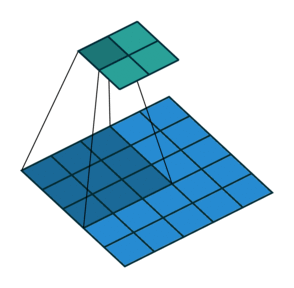with padding, s=1, f=3
with padding, s=2, f=3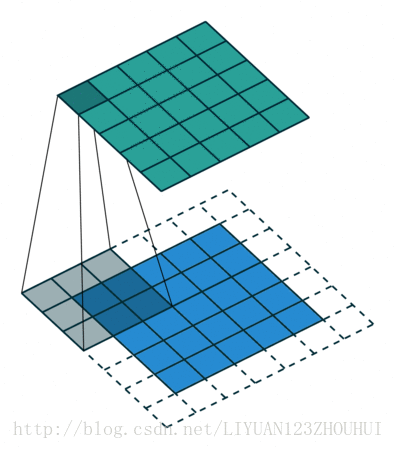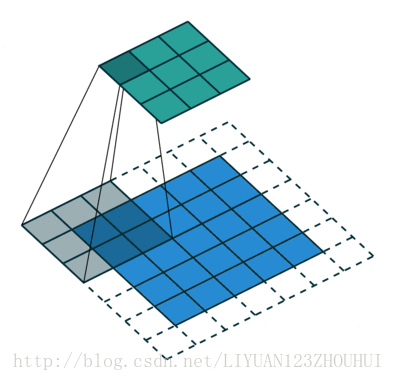3.3 完整的卷积过程
​	上面的卷积过程，没有考虑，彩色图片有rgb三维通道（Channel），如果考虑rgb通道，那么，每个通道，都需要一个卷积核：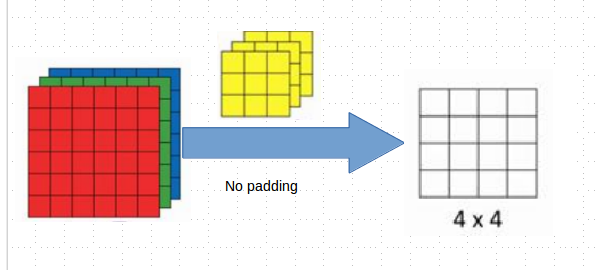​	当输入有多个通道时，我们的卷积核也需要，有同样数量的通道。以上图为例，输入有RGB三个通道，我们的就卷积核，也有三个通道，只不过计算的时候，卷积核的每个通道，在对应通道滑动（卷积核最前面的通道在输入图片的红色通道滑动，卷积核中间的通道在输入图片的绿色通道滑动，卷积核最后面的通道在输入图片的蓝色通道滑动），三个通道的计算结果相加得到输出。注意，输出只有一个通道，因为这里我们只用了一个卷积核（只不过这个卷积核有三个通道）。用下面的动图加深理解（图片来自网络，这里我们用了 2 个卷积核，每个卷积核 3 个通道，输出有 2 个通道）：​	三维展示如下（如果视频无法加载可以点击此链接查看）：
src="//player.bilibili.com/player.html?aid=23240884&cid=38697901&page=1" scrolling="no" border="0" allowfullscreen="true">
3.4 池化（Pooling)
​	池化（Pooling），有的地方也称汇聚，实际是一个下采样（Down-sample）过程。由于输入的图片尺寸可能比较大，这时候，我们需要下采样，减小图片尺寸。池化层，可以减小模型规模，提高运算速度，同时，提高所提取特征的鲁棒性。池化操作也有核大小 $f$ 和步长 $s$ 参数，参数意义和卷积相同。
​	本文主要介绍最大池化（Max Pooling）和平均池化（Average Pooling）。
​	所谓最大池化，就是对于$f\times f$大小的池化核，选取原图中数值最大的那个保留下来。比如，池化核 $2\times2$ 大小，步长为2的池化过程如下（左边是池化前，右边是池化后），对于每个池化区域都取最大值：​
​	最大池化最为常用，并且一般都取$2\times2$的池化核代大小且步长为2。
​	平均池化是取每个区域的均值（平均池化现在很少使用）: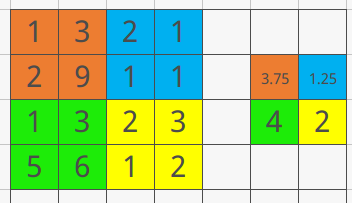3.5 激活函数
本文主要介绍 2 种激活函数，分别是$sigmoid$和$relu$函数，函数公式如下：
$sigmoid(z)=\frac{1}{1+e^{-z}}$
$relu(z)= \left\{ \begin{array}{rcl} z & z>0\\ 0&z\leq0\end{array} \right.$
函数图如下：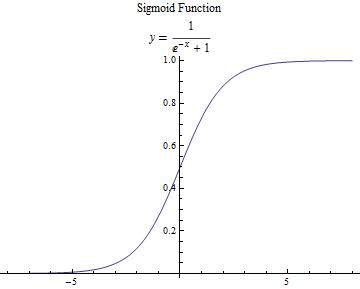$sigmoid(z)$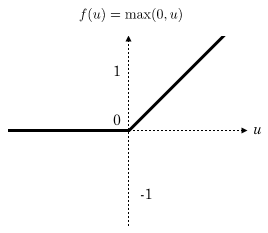$relu(z)$

补充说明
引入激活函数的目的是，在模型中引入非线性。如果没有激活函数，那么，无论你的神经网络有多少层，最终都是一个线性映射，单纯的线性映射，无法解决线性不可分问题。引入非线性可以让模型解决线性不可分问题。
一般来说，在神经网络的中间层，更加建议使用$relu$函数，两个原因：

$relu$函数计算简单，可以加快模型速度；
由于反向传播过程中，需要计算偏导数，通过求导可以得到，$sigmoid$函数导数的最大值为0.25，如果使用$sigmoid$函数的话，每一层的反向传播，都会使梯度最少变为原来的四分之一，当层数比较多的时候可能会造成梯度消失，从而模型无法收敛。

4. 卷积神经网络的前向传播过程
​	本节主要介绍，典型的卷积神经网络模型结构。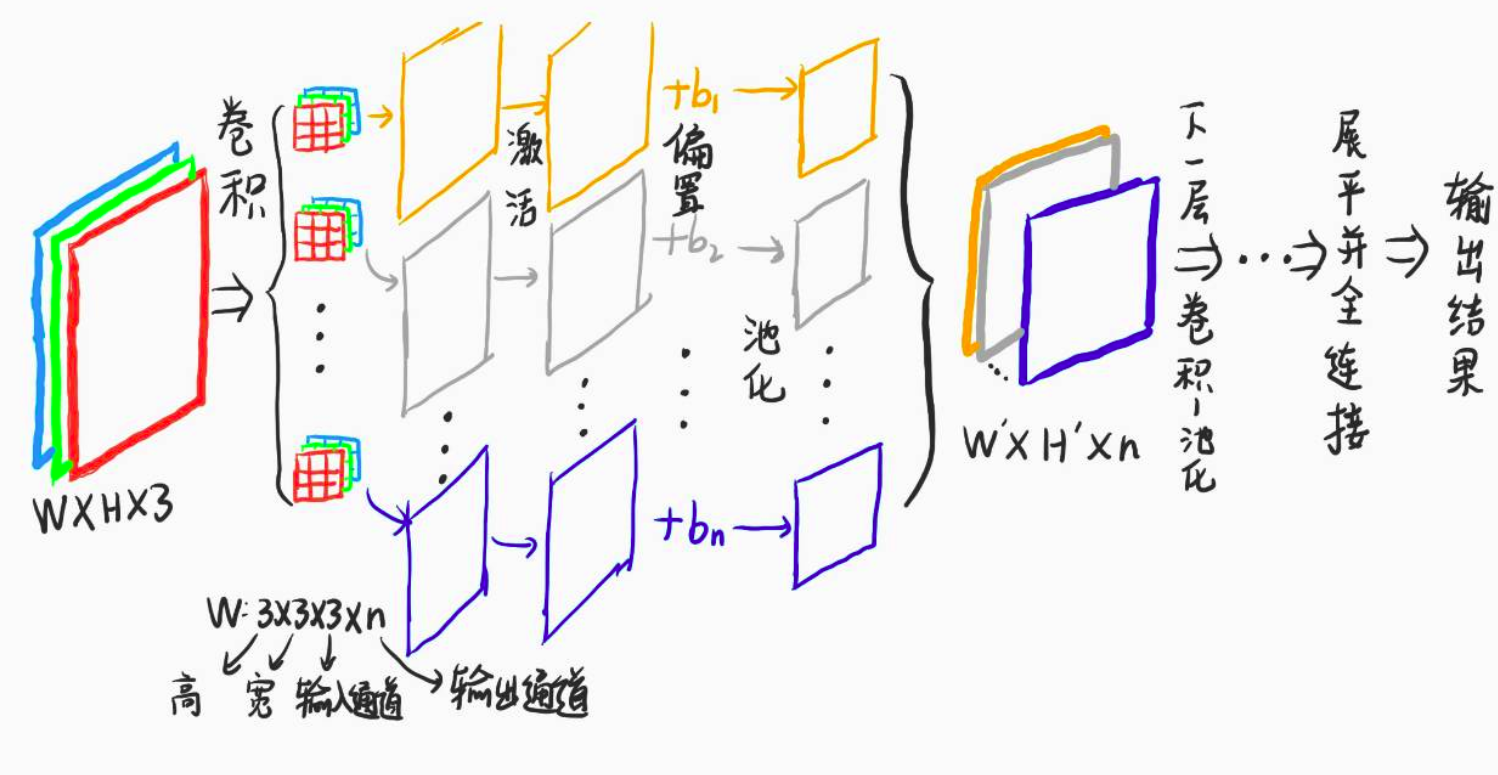​	典型的卷积网络结构，如上图所示（注意观察图中颜色对应关系）。首先输入图像，然后卷积。卷积过程采用n个卷积核，每个卷积核都有三个通道（卷积核通道数，和输入图片的通道数目相同），输出结果尺寸为$W^{'}\times H^{'}\times n$，这里的输出有n个通道，所以，下一层卷积，每个卷积核都要有n个通道；重复卷积-池化过程；模型的最后一层或两层，一般为全连接结构，输出最终结果。全连接结构部分和BP神经网络一样。最后，我们需要设计合适的损失函数，反向传播就是通过最小化损失函数，来优化网络参数，使模型达到预期结果。
​	我们把每次卷积之后，得到输出称为“特征图（Feature map）”。
​	我们定义以下符号，表示卷积网络结构（这里我尽可能模仿Tensorflow的API）：

卷积 conv2d(input, filter, strides, padding)

input：卷积输入（$H \times W \times C_{in}$，$C_{in}$指的是输入的通道数）
filter：卷积核W （$f_{height}\times f_{width}\times C_{in}\times C_{out}$）
strides：步长，一般为$1\times1\times1\times1$
padding：指明padding的算法，‘SAME’或者‘VALID’

激活 relu(conv)

conv：卷积结果

偏置相加 add(conv, b)

conv：卷积的激活输出
b：偏置系数

池化 max_pool(value, ksize, strides, padding)

value：卷积输出
ksize：池化尺寸，一般$2\times2$
strides：步长
padding：指明padding的算法，‘SAME’或者‘VALID’

5. 卷积神经网络的反向传播过程
​	终于讲到反向传播了。现在大多数深度学习框架，都帮我们做好了误差反传的工作。大部分工程师，仅仅需要构建前向传播过程，就可以了。但是，对于研究人员，有必要了解一下误差反传的原理。
​	在BP神经网络中，我们已经介绍过，全连接神经网络的反向传播过程。很多教程，在介绍卷积网络反向传播的时候，总是说，类比全连接神经网络，然后一笔带过。其实，卷积神经网络的误差反传过程，要复杂得多。原因有以下几点：

卷积神经网络的卷积核，每次滑动只与部分输入相连，具有局部映射的特点，在误差反传的时候，需要确定卷积核的局部连接方式；

卷积神经网络的池化过程，丢失了大量信息，误差反传，需要恢复这些丢失的信息；

……
  总之，卷积神经网络的误差反传过程，很复杂。之前，在写BP神经网络那篇文章的时候，推导的公式过多，导致很多读者反应，文章晦涩难懂；所以，这次我尽可能用通俗的语言来表述。


本小节的符号定义，和BP神经网络一文相同，如果出现了新的符号，会在文中对应位置说明。

5.1 卷积层的误差反传
​	假设损失函数为$Loss$, 我们希望求解，损失函数对参数 W 和偏置  b 的梯度。
​	首先，来看损失函数对卷积核 W 的梯度：
​	$l^{th}$层的卷积输出为：
$Z^{[l]}=conv2d(Z^{[l-1]}_{pool}, (W, b) , strides = 1, padding='SAME')\\ \ \ \ \ \ \ = \sum_m\sum_nW^{[l-1]}_{m^{'},n^{'}}Z^{[l-1]}_{pool\_m, n}+b^{[l]}$
​	其中，$Z^{[l-1]}_{pool\_m, n}$表示上一层池化的输出；$m^{'}$和$n^{'}$表示卷积核尺寸；$m$ 和 $n$ 表示输入的长和宽。
​	卷积层反向传播的基础，依旧是链式法则，所以，我们必须搞清楚，权重W的局部连接方式。
​	考虑前向传播过程：​	可以发现，由于卷积核在输入的特征图上滑动，所以，特征图的所有像素都参与了卷积核运算，与此对应，输出特征图的每个像素，都和卷积核 W 直接相连。
​	损失函数对参数W的梯度，根据链式法则：
$\frac{\partial Loss}{\partial W^{[l]}_{m^{'}, n^{'}}}=\sum_{i=0}^{H}\sum_{i=0}^{H}\frac{\partial Loss}{\partial Z^{[l]}_{i, j}}\frac{\partial Z^{[l]}_{i, j}}{\partial W^{[l]}_{m^{'}, n^{'}}}\\ \qquad \ =\sum_{i=0}^{H}\sum_{i=0}^{H}dZ^{[l]}_{i,j}\frac{\partial Z^{[l]}_{i, j}}{\partial W^{[l]}_{m^{'}, n^{'}}}$
​	其中，Z表示没有激活的输出，$dZ^{[l]}$表示$l^{th}$层的误差。现在我们来求解$\frac{\partial Z^{[l]}_{i, j}}{\partial W^{[l]}_{m, n}}$部分：
$\frac{\partial Z^{[l]}_{i, j}}{\partial W^{[l]}_{m^{'}, n^{'}}}=\frac{\partial}{\partial W^{[l]}_{m^{'}, n^{'}}}(\sum_m\sum_nW^{[l-1]}_{m^{'},n^{'}}Z^{[l-1]}_{pool\_m, n}+b^{[l]})\\ \qquad \ = \frac{\partial}{\partial W^{[l]}_{m^{'}, n^{'}}}(W^{[l]}_{0,0}Z^{[l-1]}_{pool\_i+0, j+0}+ . . . +W^{[l]}_{m^{'},n^{'}}Z^{[l-1]}_{pool\_i+m^{'},j+n^{'}})\\ \qquad \ =Z^{[l-1]}_{pool\_i+m^{'},j+n^{'}}$
​	综上，
$\frac{\partial Loss}{\partial W^{[l]}_{m^{'}, n^{'}}}=\sum_{i=0}^{H}\sum_{i=0}^{H}dZ^{[l]}_{i,j}\frac{\partial Z^{[l]}_{i, j}}{\partial W^{[l]}_{m^{'}, n^{'}}}\\ \qquad \quad=\sum_{i=0}^{H}\sum_{i=0}^{H}dZ^{[l]}_{i,j}Z^{[l-1]}_{pool\_i+m^{'},j+n^{'}}\\ \qquad \quad$
​	同理，可得：
$\frac{\partial Loss}{\partial b^{[l]}}=\sum_{i=0}^{H}\sum_{i=0}^{H}dZ^{[l]}_{i,j}$
​	下面来看看$dZ^{[l]}$如何求解：
$dZ^{[l]}_{i, j}=\frac{\partial Loss}{\partial Z^{[l]}_{i, j}}=\frac{\partial Loss}{\partial Z^{[l+1]}}\frac{\partial Z^{[l+1]}}{\partial Z^{[l]}}=dZ^{[l+1]}\frac{\partial Z^{[l+1]}}{\partial Z^{[l]}}$
​	也就是说我们需要求解$\frac{\partial Z^{[l+1]}}{\partial Z^{[l]}}$，所以我们需要知道在$l^{th}$层的一个像素值如何影响卷积输出：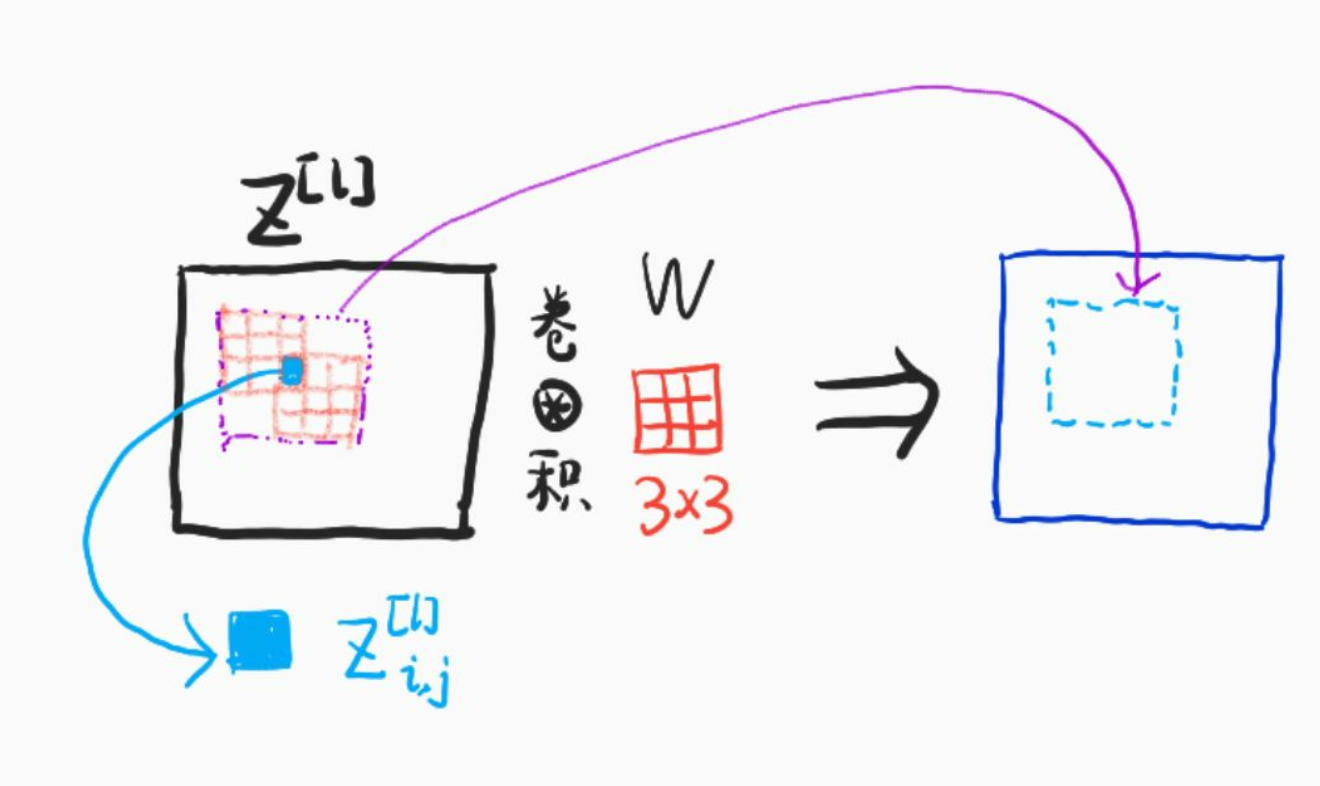​	上图说明，一个像素点，通过卷积运算，会影响输出的一个区域（输入特征图的蓝色像素点，影响了输出特征图的蓝色虚线框出的部分）。所以反向传播就是要找到，卷积核滑动过程中，一个像素点，如何通过 W 影响输出，然后逐步应用链式法则即可。
5.2 池化层的误差反传
​	池化层没有参数需要学习，但是，由于池化层的下采样操作损失了大量信息，因此，在反向传播过程中，我们需要恢复这些信息。
​	对于最大池化而言，我们需要知道在池化之前最大元素的位置：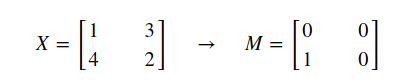​	然后，在反向传播的时候将对应值填入最大位置，这可以用池化后误差乘以M得到，这样一个数就可以恢复为$2\times 2$的矩阵，对池化层误差矩阵的每个元素都应用这样的上采样，我们就可以恢复维度信息，从而误差可以顺利反传。
​	对于平均池化而言，我们保存的是均值信息：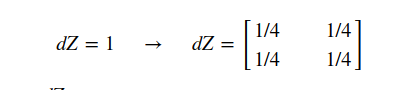​	反传过程中依旧是用池化层误差乘以dZ，将一个数恢复为$2\times 2$的矩阵即，可恢复维度信息。
6. 代码实现
​	本小节，给出了Numpy实现卷积网络的核心代码。另外，因为卷积神经网络的计算过程很复杂，因此，使用自己实现的核心代码效率很低。所以，完整模型的训练采用TensorFlow库来实现。
6.1 Numpy实现
6.1.1 卷积层前向传播
​	首先实现一个0填充的函数：
def zero_pad(X, pad):
"""零填充。

Args:
X: python numpy array of shape (m, n_H, n_W, n_C) representing a batch of m images
pad: integer, amount of padding around each image on vertical and horizontal dimensions

Returns:
X_pad -- padded image of shape (m, n_H + 2*pad, n_W + 2*pad, n_C)
"""
X_pad = np.pad(X, ((0,0),(pad,pad),(pad,pad),(0,0)), 'constant')
return X_pad

​	接下来实现一个单步卷积，即考虑全图一个切片的卷积：
def conv_single_step(a_slice_prev, W, b):
""""
Args:
a_slice_prev: slice of input data of shape (f, f, n_C_prev)
W: Weight parameters contained in a window - matrix of shape (f, f, n_C_prev)
b: Bias parameters contained in a window - matrix of shape (1, 1, 1)

Returns:
Z: a float value.
"""
s = a_slice_prev * W
Z = np.sum(s)
Z = Z + b
return Z

​	完整的一步卷积，即卷积核在输入特征图滑动：
def conv_forward(A_prev, W, b, hparameters):
"""前向传播

Args:
A_prev: output activations of the previous layer, numpy array of shape (m, n_H_prev, n_W_prev, n_C_prev)
W: Weights, numpy array of shape (f, f, n_C_prev, n_C)
b: Biases, numpy array of shape (1, 1, 1, n_C)
hparameters: a python dictionary containing "stride" and "pad"

Returns:
Z: conv output, numpy array of shape (m, n_H, n_W, n_C)
cache: cache of values needed for the conv_backward() function
"""
(m, n_H_prev, n_W_prev, n_C_prev) = A_prev.shape
(f, f, n_C_prev, n_C) = W.shape
stride = hparameters['stride']
pad = hparameters['pad']

n_H = int((n_H_prev+2*pad-f)/stride) + 1
n_W = int((n_W_prev+2*pad-f)/stride) + 1

Z = np.zeros((m,n_H,n_W,n_C))

A_prev_pad = zero_pad(A_prev, pad)

for i in range(m):
a_prev_pad = A_prev_pad[i,:,:,:]
for h in range(n_H):
for w in range(n_W):

for c in range(n_C):
vert_start = h * stride
vert_end = vert_start+f
horiz_start = w * stride
horiz_end = horiz_start+f

a_slice_prev = a_prev_pad[vert_start:vert_end,horiz_start:horiz_end, :]
Z[i, h, w, c] = conv_single_step(a_slice_prev, W[:,:,:,c], b[:,:,:,c])

cache = (A_prev, W, b, hparameters)

return Z, cache

6.1.2 卷积层反向传播
def conv_backward(dZ, cache):
"""卷积层反向传播

Args:
dZ: gradient of the cost with respect to the output of the conv layer (Z), numpy array of shape (m, n_H, n_W, n_C)
cache: cache of values needed for the conv_backward(), output of conv_forward()

Returns:
dA_prev: gradient of the cost with respect to the input of the conv layer (A_prev), numpy array of shape (m, n_H_prev, n_W_prev, n_C_prev)
dW: gradient of the cost with respect to the weights of the conv layer (W)
numpy array of shape (f, f, n_C_prev, n_C)
db: gradient of the cost with respect to the biases of the conv layer (b)
numpy array of shape (1, 1, 1, n_C)
"""
(A_prev, W, b, hparameters) = cache
(m, n_H_prev, n_W_prev, n_C_prev) = A_prev.shape
(f, f, n_C_prev, n_C) = W.shape
stride = hparameters['stride']
pad = hparameters['pad']
(m, n_H, n_W, n_C) = dZ.shape

dA_prev = np.zeros(A_prev.shape)
dW = np.zeros(W.shape)
db = np.zeros(b.shape)

A_prev_pad = zero_pad(A_prev, pad)
dA_prev_pad = zero_pad(dA_prev, pad)

for i in range(m):
a_prev_pad = A_prev_pad[i]
da_prev_pad = dA_prev_pad[i]

for h in range(n_H):
for w in range(n_W):
for c in range(n_C):
vert_start = h * stride
vert_end = vert_start + f
horiz_start = w * stride
horiz_end = horiz_start + f

a_slice = a_prev_pad[vert_start:vert_end, horiz_start:horiz_end, :]

da_prev_pad[vert_start:vert_end, horiz_start:horiz_end, :] += W[:, :, :, c] * dZ[i, h, w, c]
dW[:,:,:,c] += a_slice * dZ[i, h, w, c]
db[:,:,:,c] += dZ[i, h, w, c]

dA_prev[i, :, :, :] = da_prev_pad[pad:-pad, pad:-pad, :]

return dA_prev, dW, db

6.1.3 池化前向传播
​	池化前向传播：
def pool_forward(A_prev, hparameters, mode = "max"):
"""
Args:
A_prev: Input data, numpy array of shape (m, n_H_prev, n_W_prev, n_C_prev)
hparameters: python dictionary containing "f" and "stride"
mode: "max" or "average"

Returns:
A: output of the pool layer, a numpy array of shape (m, n_H, n_W, n_C)
cache: cache used in the backward pass of the pooling layer, contains the input and hparameters
"""
(m, n_H_prev, n_W_prev, n_C_prev) = A_prev.shape

f = hparameters["f"]
stride = hparameters["stride"]

n_H = int(1 + (n_H_prev - f) / stride)
n_W = int(1 + (n_W_prev - f) / stride)
n_C = n_C_prev

A = np.zeros((m, n_H, n_W, n_C))

for i in range(m):
for h in range(n_H):
for w in range(n_W):
for c in range (n_C):
vert_start = h*stride
vert_end = vert_start+f
horiz_start = w*stride
horiz_end = horiz_start+f

a_prev_slice = A_prev[i, vert_start:vert_end, horiz_start:horiz_end, c]

if mode == "max":
A[i, h, w, c] = np.max(a_prev_slice)
elif mode == "average":
A[i, h, w, c] = np.mean(a_prev_slice)

cache = (A_prev, hparameters)

return A, cache

6.1.4 池化反向传播
​	首先，对于最大池化，我们需要记录前向传播过程中最大值在哪个位置：
def create_mask_from_window(x):
"""
Args:
x: Array of shape (f, f)

Returns:
maskL: Array of the same shape as window, contains a True at the position corresponding to the max entry of x.
"""
mask = x == np.max(x)

return mask

​	对于平均池化，我们需要把误差平均分到每个位置：
def distribute_value(dz, shape):
"""
Args:
dz: input scalar
shape: the shape (n_H, n_W) of the output matrix for which we want to distribute the value of dz

Returns:
a: Array of size (n_H, n_W) for which we distributed the value of dz
"""
(n_H, n_W) = shape
average = dz / (n_H * n_W)
a = np.ones((n_H, n_W)) * average
return a

​	接下来，我们实现完整的池化层反向传播过程：
def pool_backward(dA, cache, mode = "max"):
"""
Args:
dA: gradient of cost with respect to the output of the pooling layer, same shape as A
cache: cache output from the forward pass of the pooling layer, contains the layer's input and hparameters
mode: the pooling mode you would like to use, defined as a string ("max" or "average")

Returns:
dA_prev: gradient of cost with respect to the input of the pooling layer, same shape as A_prev
"""
(A_prev, hparameters) = cache
stride = hparameters['stride']
f = hparameters['f']
m, n_H_prev, n_W_prev, n_C_prev = A_prev.shape
m, n_H, n_W, n_C = dA.shape

dA_prev = np.zeros(A_prev.shape)

for i in range(m):
a_prev = A_prev[i]
for h in range(n_H):
for w in range(n_W):
for c in range(n_C):
vert_start = h * stride
vert_end = vert_start + f
horiz_start = w * stride
horiz_end = horiz_start + f

if mode == "max":
a_prev_slice = a_prev[vert_start:vert_end, horiz_start:horiz_end, c]
mask = create_mask_from_window(a_prev_slice)
dA_prev[i, vert_start: vert_end, horiz_start: horiz_end, c] += np.multiply(mask, dA[i, h, w, c])

elif mode == "average":
da = dA[i, h, w, c]
shape = (f, f)
dA_prev[i, vert_start:vert_end, horiz_start:horiz_end, c] += distribute_value(da, shape)

return dA_prev

6.2 Tensorflow框架实现
​	由于自己实现的卷积网络核心代码，计算速度过慢（只能在CPU运行，且包含太多for循环，效率过低），所以最终决定用TensorFlow来完成一个测试模型。
​	我们选用TensorFlow实现一个CIFAR-10数据集分类的例子。CIFAR-10是一个用于图片分类的数据集。此数据集由60000张图片组成，包涵10类。其中50000张图片用于训练，10000张图片用于测试。
​	本文不会具体介绍TensorFlow的详细用法，如果需要，可以参考TensoFlow官网教程，或者gitchat也有人写过一些入门文章。TensorFlow实现深度学习模型一般包涵以下步骤：

定义占位符

构建模型的前向传播过程

定义损失函数

指定优化方法

运行优化方法最小化损失函数
我使用jupyter notebook编程环境。首先，导入必须的包，导入数据文件：

import os
import tensorflow as tf
import numpy as np
import math
import timeit
import matplotlib.pyplot as plt
gpu_no = '0'  # or '1'

os.environ["CUDA_VISIBLE_DEVICES"] = gpu_no
config = tf.ConfigProto()

config.gpu_options.allow_growth = True

%matplotlib inline

def load_cifar10(num_training=49000, num_validation=1000, num_test=10000):
"""
导入CIFAR数据集。
"""
cifar10 = tf.keras.datasets.cifar10.load_data()
(X_train, y_train), (X_test, y_test) = cifar10
X_train = np.asarray(X_train, dtype=np.float32)
y_train = np.asarray(y_train, dtype=np.int32).flatten()
X_test = np.asarray(X_test, dtype=np.float32)
y_test = np.asarray(y_test, dtype=np.int32).flatten()

mask = range(num_training, num_training + num_validation)
X_val = X_train[mask]
y_val = y_train[mask]
mask = range(num_training)
X_train = X_train[mask]
y_train = y_train[mask]
mask = range(num_test)
X_test = X_test[mask]
y_test = y_test[mask]

mean_pixel = X_train.mean(axis=(0, 1, 2), keepdims=True)
std_pixel = X_train.std(axis=(0, 1, 2), keepdims=True)
X_train = (X_train - mean_pixel) / std_pixel
X_val = (X_val - mean_pixel) / std_pixel
X_test = (X_test - mean_pixel) / std_pixel

return X_train, y_train, X_val, y_val, X_test, y_test

X_train, y_train, X_val, y_val, X_test, y_test = load_cifar10()

class Dataset(object):
def __init__(self, X, y, batch_size, shuffle=False):
assert X.shape == y.shape, 'Got different numbers of data and labels'
self.X, self.y = X, y
self.batch_size, self.shuffle = batch_size, shuffle

def __iter__(self):
N, B = self.X.shape, self.batch_size
idxs = np.arange(N)
if self.shuffle:
np.random.shuffle(idxs)
return iter((self.X[i:i+B], self.y[i:i+B]) for i in range(0, N, B))

train_dset = Dataset(X_train, y_train, batch_size=64, shuffle=True)
val_dset = Dataset(X_val, y_val, batch_size=64, shuffle=False)
test_dset = Dataset(X_test, y_test, batch_size=64)

​	构建计算图（前向传播过程）：
def conv2d(x, scope, W_shape, b_shape, stddev = 5e-2, stride = [1, 1, 1, 1], lambda_ = 0.005):
"""
定义卷积层
"""
with tf.variable_scope(scope, reuse = tf.AUTO_REUSE) as scope:

initializer = tf.truncated_normal_initializer(stddev=stddev, dtype=tf.float32)

W = tf.get_variable('W', W_shape, initializer=initializer, dtype=tf.float32)
b = tf.get_variable('b', b_shape, initializer=tf.constant_initializer(0.0), dtype=tf.float32)

tf.add_to_collection("l2_loss", tf.nn.l2_loss(W)*lambda_)
conv = tf.nn.conv2d(x, W, stride, padding='SAME')
conv = tf.add(tf.nn.relu(conv), b)
return conv

def max_pool(x, ksize = [1, 2, 2,1], stride = [1, 2, 2, 1]):
"""
池化层
"""
out = tf.nn.max_pool(x, ksize, stride, padding='SAME')
return out

def fully_connect(x, scope, indim, outdim, stddev=5e-2):
"""
全连接层
"""
with tf.variable_scope(scope, reuse=tf.AUTO_REUSE) as scope:
reshape = tf.reshape(x, [tf.shape(x), -1])

initializer = tf.truncated_normal_initializer(stddev=stddev, dtype=tf.float32)

W = tf.get_variable('W', [indim, outdim], initializer=initializer, dtype=tf.float32)
b = tf.get_variable('b', [outdim], initializer=tf.constant_initializer(0.0), dtype=tf.float32)
tf.add_to_collection("l2_loss", tf.nn.l2_loss(W)*0.004)
fc_out = tf.nn.relu(tf.matmul(reshape, W) + b)
return fc_out

def build_model(images):
"""
构建完整模型
conv1->relu->pool->norm->conv2->pool->norm->fully1->relu->fully2->relu->softmax
"""
temp_conv = conv2d(images, "conv1", [5, 5, 3, 64], , stddev = 5e-2)
temp_pool = max_pool(temp_conv, [1, 3, 3, 1])
temp_norm = tf.nn.lrn(temp_pool, 4, bias=1.0, alpha=0.001/9, beta=0.75)
temp_conv = conv2d(temp_norm, "conv2", [5, 5, 64, 64], , stddev = 5e-2)
temp_pool = max_pool(temp_conv)
temp_norm = tf.nn.lrn(temp_pool, 4, bias=1.0, alpha=0.001/9, beta=0.75)

fc = fully_connect(temp_pool, 'fully1', 8*8*64, 256)
fc = fully_connect(fc, 'fully2', 256, 10)
out = tf.nn.softmax(fc)

return out

def loss(y_pred, y):
"""
损失函数
"""
labels = tf.reshape(tf.one_hot(tf.reshape(tf.cast(y, dtype=tf.int64),[1, -1]), 10), [-1, 10])
softmax_loss = tf.reduce_mean(tf.nn.softmax_cross_entropy_with_logits_v2(logits=y_pred,
labels=labels))
loss = tf.reduce_sum(tf.get_collection("l2_loss")) + softmax_loss
#loss = softmax_loss
return loss

def accuracy(y_pred, y):
"""
准确率计算
"""
y_pred = tf.argmax(y_pred, axis=1)
y = tf.reshape(tf.cast(y,tf.int64),[1, -1])
acc = tf.reduce_mean(tf.cast(tf.equal(y_pred,y), tf.float32))
return acc

def train(train_dst, sess, epochs=100):
"""
训练图
"""
train_accs = []
val_accs = []

#占位符
images = tf.placeholder(tf.float32, [None, 32, 32, 3])
y_label = tf.placeholder(tf.float32, [None, 1])

#模型
model = build_model(images)
y_pred = model
#准确率
acc = accuracy(y_pred, y_label)
#损失函数
losses = loss(y_pred, y_label)

#优化器
with tf.variable_scope('opt', reuse = tf.AUTO_REUSE):
opt = tf.train.AdamOptimizer(learning_rate=0.0001, use_locking=True)
train_step = opt.minimize(losses)

#参数初始化
init = tf.global_variables_initializer()
sess.run(init)

for epoch in range(epochs):
#迭代训练
print("****************epoch "+str(epoch))
l = 0
for (X, y) in train_dst:
y_pred_ = sess.run(model, feed_dict={images:X})
#print(y_pred.shape,type(y_pred))
l = sess.run(losses,  feed_dict={images:X, y_label: y.reshape((-1, 1))} )

train_acc = sess.run(acc, feed_dict={images:X, y_label: y.reshape((-1, 1))})
train_accs.append(train_acc)

sess.run(train_step, feed_dict={images:X, y_label:y.reshape((-1, 1))})

print("loss: " +str(l))

if epoch%1==0:

print("train_acc: "+str(train_acc))
val_acc = 0
for i, (X_v, y_v) in enumerate(val_dset):

y_pred_val = sess.run(model,feed_dict={images: X_v})
val_acc += sess.run(acc, feed_dict={images: X_v, y_label: y_v.reshape((-1, 1))})
val_acc /= (i+1)
val_accs.append(val_acc)
print("val_acc: "+ str(val_acc))
#测试集
test_acc = 0
for i, (X_t, y_t) in enumerate(test_dset):
y_pred_test = sess.run(model, feed_dict={images: X_t})
test_acc += sess.run(acc, feed_dict={images: X_t, y_label:y_t.reshape((-1, 1))})
test_acc /= (i+1)
print("%%%%%%%%%%%%% Test accuracy")
print(test_acc)
return train_accs,